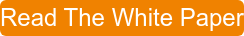Bob Bickler

When developing reversed-phase flash chromatography methods it is important to understand the impact that a change in solvent ratio has on compound retention and, therefore, separation performance. Unlike normal-phase chromatography where you can optimize separations using TLC and a wide variety of solvents and solvent ratios, reversed-phase limits you to 3 to 4 solvents, including water, using either HPLC or small flash columns for method development. Those solvents include:

• Water
• Methanol
• Acetonitrile
• Tetrahydrofuran

One of the most frequently asked question I am asked is with what % organic do I begin my method development? This is a pertinent question as a 0-100% gradient is not necessarily a good method or even a good starting point.

You do need to consider sample solubility when determining the gradient starting point or % B. If your product is water or alcohol soluble, start with a low % B (e.g. 10%), if organic soluble (hexane, DCM, EtOAc, acetone), start with a high % B (e.g. 40%) and ramp 50% B over 10 CV, then adjust accordingly as outlined below.

I have posted on this previously but want to address another aspect of method development – predicting elution volume or time based on the amount of compound retention shift you see with a change in strong solvent %, e.g. % methanol or acetonitrile. Rather than just tell you, I believe it important to show you the relationship of compound retention and % B both graphically and in a table.

To illustrate the % B change impact on compound retention I set up a series of experiments with a gradient slope of 5%B/column volume (50% increase in B over 10 column volumes). Changing gradient slope is another variable in method development to be covered in a different post.

This 5% slope paradigm enabled me to run the following 10 column volume (CV) acetonitrile gradient experiments to determine the correlation.

• 10% to 60% B
• 20% to 70% B
• 30% to 80% B
• 40% to 90% B

My sample was a 1:1 mix of methyl paraben (MP) and butyl paraben (BP) in acetone. My column was a 12-gram Biotage® Sfär C18, the flash system used was a Biotage® Selekt at 30 mL/min.

The four runs provided useful data points and provided me enough information to determine their correlation. The results show a linear correlation of compound retention and % B, Table 1, which is better seen in the graph in Figure 1.

Table 1. Elution volumes for methyl paraben (MP) and butyl paraben (BP) with different water/acetonitrile gradient starting points.

Start% B     MP (mL)     BP (mL)
10                    118              176
20                    82               141
30                    49               108
40                    31                69Figure 1. Compound retention change with increasing % B shows a nearly linear correlation for both compounds.

With correlation factors of essentially 1, we can determine that the retention vs. % B slope is essentially linear. We also now know that we can use the slopes for each of the two compounds to predict their elution volume for any other 5% slope gradient starting between 10% and 40% B.

Putting this in practice, we know the methyl paraben slope is ~30 mL/10% B change or 3 mL/1% change and the butyl paraben slope per 10% change in B is ~36 mL (3.6 mL/1% change). So, for a 25% to 75% acetonitrile gradient, the predicted elution volumes for methyl paraben and butyl paraben are 64 mL and 126 mL, respectively.

How did I get there?

Methyl paraben -

Its slope = 3 mL/%; Slope (3 mL/%) x 5% change in gradient starting % (30% B – 25% B = 5%) = 3 mL/% x 5% = 15 mL; 15 mL + 49 mL (elution volume at 30% B) = 64 mL

Butyl paraben -

Slope = 3.6 mL/%; Slope (3.6 mL/%) x 5% change in gradient starting % (30% B – 25% B = 5%) = 2.6 mL/% x 5% = 18 mL; 18 mL + 108 mL (elution volume at 30% B) = 126 mL

The results show the prediction was very close and provided actual elution volumes of 62 mL and 123 mL, Figure 2.Figure 2. Chromatogram of methyl and butyl paraben in a 25-75% acetonitrile gradient shows good correlation to predicted elution volumes.

Methanol/water gradients will also show linearity within specific starting points as well, when gradient slope is maintained.

So, when you are developing a reversed-phase method and your compounds elute too late or too early, consider changing the starting and ending % B while maintaining the gradient slope. Run a 10-60% gradient and a 40-90% gradient. If your target compound is not eluting and separating as desired, determine the retention slope and choose a starting % B which provides the needed retention.

For more flash chromatography information download our whitepaper - Successful Flash Purification.